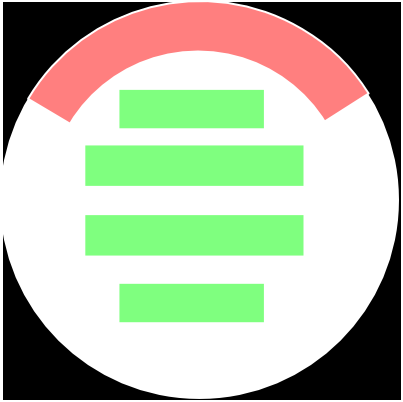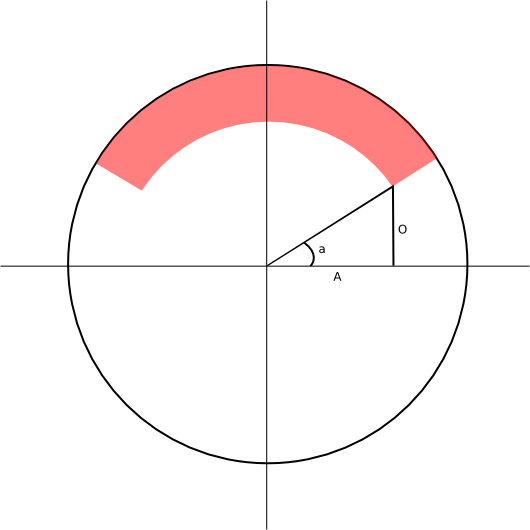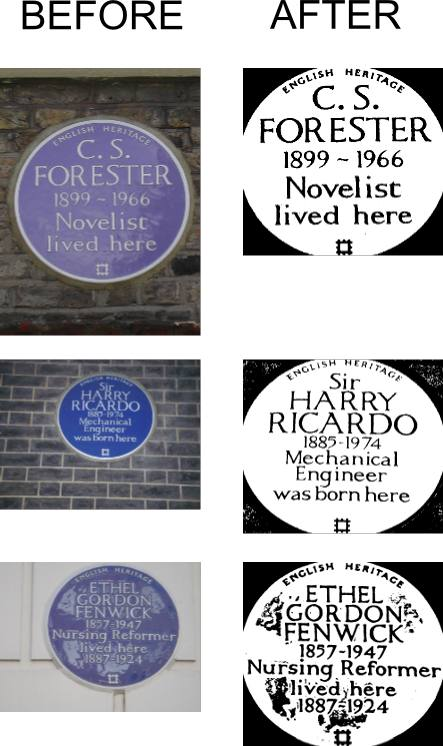## Images and Vision in Python: Slides from talk at Python Edinburgh Mini-Conf 2011

Last weekend the Python Edinburgh users group hosted a mini-conference. Saturday morning was kicked off with a series of talks followed by sessions introducing and then focusing on contributing to django prior to sprints which really got going on the Sunday.

The slides for my talk on, "Images and Vision in Python" are now available in pdf format here.

The slide deck I used is relatively lightweight with my focus being on demonstrating using the different packages available. The code I went through is below.

```from PIL import Image

#Open an image and show it
pil1 = Image.open('filename')
pil1.show()

#Get its size
pil1.size
#Resize
pil1s = pil1.resize((100,100))
#or - thumbnail
pil1.thumbnail((100,100), Image.ANTIALIAS)

#New image
bg = Image.new('RGB', (500,500), '#ffffff')

#Two ways of accessing the pixels

for a in range(100, 200):
for b in range(100,110):
pix[a,b] = (0,0,255)
bg.show()

#Drawing shapes is slightly more involved
from PIL import ImageDraw
draw = ImageDraw.Draw(bg)
draw.ellipse((300,300,320,320), fill='#ff0000')
bg.show()

from PIL import ImageFont
font = ImageFont.truetype("/usr/share/fonts/truetype/freefont/FreeSerif.ttf", 72)
draw.text((10,10), "Hello", font=font, fill='#00ff00')
bg.show()

#Demo's for vision
from scipy import ndimage
import mahotas

#Create a sample image
v1 = np.zeros((10,10), bool)
v1[1:4,1:4] = True
v1[4:7,2:6] = True
imshow(v1, interpolation="Nearest")
imshow(mahotas.dilate(v1), interpolation="Nearest")
imshow(mahotas.erode(v1), interpolation="Nearest")
imshow(mahotas.thin(v1), interpolation="Nearest")

#Opening, closing and top-hat as combinations of dilate and erode

#Labeling
v1[8:,8:] = True
imshow(v1)
labeled, nr_obj = ndimage.label(v1)
nr_obj
imshow(labeled, interpolation="Nearest")
pylab.jet()

#Thresholding
#Convert a grayscale image to a binary image
T = mahotas.otsu(v2)
imshow(v2)
imshow(v2 > T)

#Distance Transforms
dist = mahotas.distance(v2 > T)
imshow(dist)
```

## AI Cookbook Competition - Month Three

A little over two months ago I wrote about the first round of the AI cookbook competition. Since then there have been two further rounds and a considerable amount of further progress. For the latest round I was able to get the error score down to 10.867 using an additional image pre-processing step and then a variety of text clean-up improvements.

#### Image Pre-processing

Ian, who writes the AI Cookbook, had the theory that the curved text present at the top of many of the plaques in the test set were causing tesseract, our OCR software of choice, significant problems in transcribing the main text. If we could automatically recognise the curved text and block it out the transcription should be significantly improved. In the diagram below the text we want to be transcribed is in green and the text we don't want is in red.I couldn't think of a good method to actually recognise the curved text at the top so decided to use a 'dumb' approach. The curved text is in the same place on all the plaques so I built a system to apply the same mask to all the images. To do this I went back to what I could still remember from high school math lessons. To the probable delight of my old math teachers I quickly had some working code. The code I wrote cycles through all the pixels in the image and converts them to a distance and angle relative to the centre of the image. This process is hopefully easier to visualise in the image below. The distance is simple enough to calculate as we're dealing with a right-angle triangle; we simply square the x and y values, add them together and take the square root. The angle is a little trickier. The y-value represents the opposite length of the triangle and the x-value represents the adjacent length so from the mnemonic SOH CAH TOA we known the angle will be tan-1 (O/A). Knowing that we can then apply our rules for distance and angle.#### Text Clean-up

The text clean-up was lots of little steps. Briefly I've,

1. Made various improvements to the regexes for cleaning up the years
2. Converted any instances of 'vv' (two v's) to 'w' (one w)
3. Switched 0 (zero) to o (letter o) in words
4. Removed any one/two character tokens from the end of the string
5. Improved the selection of suggestions from the spell checker
6. Broken up long words to see if a valid word can be found in the two halves
7. Changed "s to 's
8. Improved correction for endings where the ending is lived|worked|died here and the spelling checker returns bad results
9. Removed any words containing three of lowercase, uppercase, digits and punctuation.

The regex for that last item is something of a monstrosity and as I'm far from an expert it wouldn't surprise me if it doesn't entirely do what I think it does. I've used whitespace to make it slightly easier to follow. Each line represents a sub-expression, if any sub-expression matches the string then the expression as a whole is considered to match. Each line matches a different combination of three from digits, lowercase, uppercase and punctuation. The .+ at the end means we match one or more of any character. The expressions in brackets starting with a question mark are look ahead assertions. The .+ still matches any character but the look ahead assertions state that at least one of the characters matched must be a digit for instance. It doesn't matter in what order the characters are present as long as they are present. If you suspect there is a flaw in the pattern or know some way to simplify it then I would really appreciate a quick note in the comments field below.

```re.compile(r""" #matching a combination of digits, lowercase, uppercase and punctuation
((?=.*\d)(?=.*[a-z])(?=.*['"-,\.]).+| #d,l,p
(?=.*[A-Z])(?=.*[a-z])(?=.*['"-,\.]).+| #u,l,p
(?=.*\d)(?=.*[A-Z])(?=.*[a-z]).+| #d,u,l
(?=.*\d)(?=.*[A-Z])(?=.*['"-,\.]).+ #u,p,d
)""", re.VERBOSE)
```

That's all for now. I believe Ian is planning to run the competition for a further month and there are still considerable improvements to be made so it would be great to see more people taking part.

## AI cookbook competition - transcription for the openplaques project

Ian Ozsvald over at aicookbook has been doing some work using optical character recognition (OCR) to transcribe plaques for the openplaques group. His write-ups have been interesting so when he posted a challenge to the community to improve on his demo code I decided to give it a try.

The demo code was very much a proof of principle and its score of 709.3 was easy to beat. I managed to quickly get the score down to 44 and with a little more work reached 33.4. The score is a Levenshtein distance metric so the lower the better. I was hoping to get below 30 but in the end just didn't have time. I suspect it wouldn't take a lot of work to improve on my score. Here's what I've done so far . . .

### Configure the system

All the work I've done was on an Ubuntu 10.04 installation and the instructions which follow will only deal with this environment. Beyond the base install I use three different packages:

Python Image Library
Used for pre-processing the images before submitting to tesseract
Tesseract
The OCR software used
Enchant spellchecker
Used for cleaning up the transcribed text

Their installation is straightforward using apt-get

```\$ sudo apt-get install python-imaging python-enchant tesseract-ocr tesseract-ocr-eng
```

### Fetch images

The demo code written by Ian (available here) includes a script to fetch the images from flickr. It's as simple as running the following

```\$ python get_plaques.py easy_blue_plaques.csv
```

Once the images are downloaded I suggest you go ahead and run the demo transcribing script. Again it's nice and simple

```\$ python plaque_transcribe_demo.py easy_blue_plaques.csv
```

Then you can calculate the score using

```\$ python summarise_results.py results.csv
```

### Improving transcription

Ian had posted a number of good suggestions on the wiki for how to improve the transcription quality. I used four approaches:

Image preprocessing
Cropping the image and converting to black and white takes the score from 782 (the demo code produced a higher score on my system than it did for Ian) to 44.6
Restricting the characters tesseract will return
By restricting the character set used by tesseract to alphanumeric characters and a limited selection of punctuation characters further lowered the score from 44.6 to 35.7
Spell checking
Running the results from tesseract through a spell checker and filtering out some common errors brought the score down to 33.4

I'll post the entire script at the bottom of this post but want to highlight a few of the key elements first.

The first stage of cropping the image on the plaque is handled by the function crop_to_plaque which expects a python image library image object. The function then reduces the size of the image to speed up processing before looking for blue pixels. A blue pixel is assumed to be any pixel where the value of the blue channel is 20% higher than both the red and green channels. The number of blue pixels in each row and column of the image is counted and then the image is cropped down to the rows and columns where the number of blue pixels is greater than 15% of the height and width of the image. This value is based solely on experimentation and seemed to give good results for this selection of plaques.

The next stage of converting the image to black and white is handled by the function convert_to_bandl which again expects a python image library image object. The function converts any blue pixels to white and all other pixels to black. Ian has pointed out that this approach might be overly stringent and I might get better results using some grey as well. The result of running these two functions on three of the plaques is shown below.The next step was limiting the character set used by tesseract. The easiest way to do this is to create a file in /usr/share/tesseract-ocr/tessdata/configs/ which I called goodchars with the following content.

```0123456789abcdefghijklmnopqrstuvwxyzABCDEFGHIJKLMNOPQRSTUVWXYZ.,()-"
```

That selection of characters seems to include all the characters present in the plaques. To use this limited character set the call to tesseract needs to be altered to

```cmd = 'tesseract %s %s -l eng nobatch goodchars' % (filename_tif, filename_base)
```

Finally I perform a bunch of small clean up tasks. Firstly I fix the year ranges which frequently had extra spaces inserted and occasionally 1s appeared as i or l and 3 appeared as a parenthesis. These were fixed by a couple of regular expressions including one callback function (clean_years). Then I seperate the transcription out into individual words and fix a number of more issues including lone characters and duplicated characters before checking the spelling on any words of more than two characters.

### Where next?

There is still lots of 'low hanging fruit' on this problem. At the moment the curved text at the top of the plaque and the small symbol at the bottom of the plaques is handled badly and I think the bad characters at the beginning and end of the transcriptions could be easily stripped out. The spelling corrections I make do overall reduce the error but they introduce some new errors. I suspect by being more selective in where spelling checks are made some of these introduced errors could be removed.

### The entire script

```import os
import sys
import csv
import urllib
from PIL import Image # http://www.pythonware.com/products/pil/
import ImageFilter
import enchant
import re

# This recognition system depends on:
# version 2.04, it must be installed and compiled already

# plaque_transcribe_test5.py
# run it with 'cmdline> python plaque_transcribe_test5.py easy_blue_plaques.csv'
# and it'll:
# 1) send images to tesseract
# 2) read in the transcribed text file
# 3) convert the text to lowercase
# 4) use a Levenshtein error metric to compare the recognised text with the
# human supplied transcription (in the plaques list below)
# 5) write error to file

# For more details see:
# http://aicookbook.com/wiki/Automatic_plaque_transcription

"""build plaques structure from CSV file"""
plaques = []
for row in plqs:
image_url = row
text = row
# ignore id (0) and plaque url (3) for now
last_slash = image_url.rfind('/')
filename = image_url[last_slash+1:]
filename_base = os.path.splitext(filename) # turn 'abc.jpg' into 'abc'
filename = filename_base + '.tif'
root_url = image_url[:last_slash+1]
plaque = [root_url, filename, text]
plaques.append(plaque)
return plaques

def levenshtein(a,b):
"""Calculates the Levenshtein distance between a and b
Taken from: http://hetland.org/coding/python/levenshtein.py"""
n, m = len(a), len(b)
if n > m:
# Make sure n <= m, to use O(min(n,m)) space
a,b = b,a
n,m = m,n

current = range(n+1)
for i in range(1,m+1):
previous, current = current, [i]+*n
for j in range(1,n+1):
change = previous[j-1]
if a[j-1] != b[i-1]:
change = change + 1

return current[n]

def transcribe_simple(filename):
"""Convert image to TIF, send to tesseract, read the file back, clean and
return"""
# read in original image, save as .tif for tesseract
im = Image.open(filename)
filename_base = os.path.splitext(filename) # turn 'abc.jpg' into 'abc'

#Enhance contrast
#contraster = ImageEnhance.Contrast(im)
#im = contraster.enhance(3.0)
im = crop_to_plaque(im)
im = convert_to_bandl(im)

filename_tif = 'processed' + filename_base + '.tif'
im.save(filename_tif, 'TIFF')

# call tesseract, read the resulting .txt file back in
cmd = 'tesseract %s %s -l eng nobatch goodchars' % (filename_tif, filename_base)
print "Executing:", cmd
os.system(cmd)
input_filename = filename_base + '.txt'
input_file = open(input_filename)
line = " ".join([x.strip() for x in lines])
input_file.close()
# delete the output from tesseract
os.remove(input_filename)

# convert line to lowercase
transcription = line.lower()

#Remove gaps in year ranges
transcription = re.sub(r"(\d+)\s*-\s*(\d+)", r"\1-\2", transcription)
transcription = re.sub(r"([0-9il\)]{4})", clean_years, transcription)

#Separate words
d = enchant.Dict("en_GB")
newtokens = []
print 'Prior to post-processing: ', transcription
tokens = transcription.split(" ")
for token in tokens:
if (token == 'i') or (token == 'l') or (token == '-'):
pass
elif token == '""':
newtokens.append('"')
elif token == '--':
newtokens.append('-')
elif len(token) > 2:
if d.check(token):
#Token is a valid word
newtokens.append(token)
else:
#Token is not a valid word
suggestions = d.suggest(token)
if len(suggestions) > 0:
#If the spell check has suggestions take the first one
newtokens.append(suggestions)
else:
newtokens.append(token)
else:
newtokens.append(token)

transcription = ' '.join(newtokens)

return transcription

def clean_years (m):
digits = m.group(1)
year = []
for digit in digits:
if digit == 'l':
year.append('1')
elif digit == 'i':
year.append('1')
elif digit == ')':
year.append('3')
else:
year.append(digit)
return ''.join(year)

def crop_to_plaque (srcim):

scale = 0.25
wkim = srcim.resize((int(srcim.size * scale), int(srcim.size * scale)))
wkim = wkim.filter(ImageFilter.BLUR)
#wkim.show()

width = wkim.size
height = wkim.size

#result = wkim.copy();
highlight_color = (255, 128, 128)
R,G,B = 0,1,2
lrrange = {}
for x in range(width):
lrrange[x] = 0
tbrange = {}
for y in range(height):
tbrange[y] = 0

for x in range(width):
for y in range(height):
point = (x,y)
pixel = wkim.getpixel(point)
if (pixel[B] > pixel[R] * 1.2) and (pixel[B] > pixel[G] * 1.2):
lrrange[x] += 1
tbrange[y] += 1
#result.putpixel(point, highlight_color)

#result.show();

left = 0
right = 0
cutoff = 0.15
for x in range(width):
if (lrrange[x] > cutoff * height) and (left == 0):
left = x
if lrrange[x] > cutoff * height:
right = x

top = 0
bottom = 0
for y in range(height):
if (tbrange[y] > cutoff * width) and (top == 0):
top = y
if tbrange[y] > cutoff * width:
bottom = y

left = int(left / scale)
right = int(right / scale)
top = int(top / scale)
bottom = int(bottom / scale)

box = (left, top, right, bottom)
region = srcim.crop(box)
#region.show()

return region

def convert_to_bandl (im):
width = im.size
height = im.size

white = (255, 255, 255)
black = (0, 0, 0)
R,G,B = 0,1,2

for x in range(width):
for y in range(height):
point = (x,y)
pixel = im.getpixel(point)
if (pixel[B] > pixel[R] * 1.2) and (pixel[B] > pixel[G] * 1.2):
im.putpixel(point, white)
else:
im.putpixel(point, black)
#im.show()
return im

if __name__ == '__main__':
argc = len(sys.argv)
if argc != 2:
print "Usage: python plaque_transcribe_demo.py plaques.csv (e.g. \
easy_blue_plaques.csv)"
else:

results = open('results.csv', 'w')

for root_url, filename, text in plaques:
print "----"
print "Working on:", filename
transcription = transcribe_simple(filename)
print "Transcription: ", transcription
print "Text: ", text
error = levenshtein(text, transcription)
assert isinstance(error, int)
print "Error metric:", error
results.write('%s,%d\n' % (filename, error))
results.flush()
results.close()
```